# Circle

What is the radius of the circle whose perimeter is 10 mm?

r =  1.59 mm

### Step-by-step explanation:We will be pleased if You send us any improvements to this math problem. Thank you!#### You need to know the following knowledge to solve this word math problem:

We encourage you to watch this tutorial video on this math problem:

## Related math problems and questions:

• Circle from string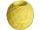Martin has a long 628 mm string. He makes a circle from it. Calculate the radius of the circle.
• Rectangle - desc circle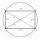Length of the sides of the rectangle are at a ratio 1: 3 . Radius of the circle circumscribed to rectangle is 10 cm. Calculate the rectangle's perimeter.
• Circle and angleWhat is the length of the arc of a circle with radius r = 207 mm with cental angle 5.33 rad?
• Diameter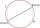If the endpoints of a diameter of a circle are A(10, -1) and B (3, 10), what is the radius of the circle?
• RadiusDetermine the radius of the circle, if its perimeter and area is the same number.
• Rectangle and circle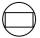The rectangle ABCD has side lengths a = 40 mm and b = 30 mm and is circumscribed by a circle k. Calculate approximately how many cm is circle long.
• Perimeter of the circleCalculate the perimeter of the circle in dm, whose radius equals the side of the square containing 0.49 dm2?
• Intersections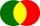How many intersections have circles with radius 16 mm and 15 mm, if the distance of their centers is 16 mm.
• Circle - simpleThe circumference of a circle is 198 mm. How long in mm is its diameter?
• Radius of the circleCalculate the radius of the circle whose length is 107 cm larger than its diameter
• Chord 3What is the radius of the circle where the chord is 2/3 of the radius from the center and has a length of 10 cm?
• Hexagon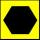Draw a regular hexagon inscribed in a circle with radius r=8 cm. What is its perimeter?
• Circumscribed circle to squareFind the length of a circle circumscribing a square of side 10 cm. Compare it to the perimeter of this square.
• Circle - easy 2The circle has a radius 6 cm. Calculate:
• Circle r,DCalculate the diameter and radius of the circle if it has length 26.59 cm.
• Square and circle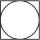Into square is inscribed circle with diameter 10 cm.What is difference between circumference square and circle?
• Rope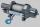How many meters of rope 10 mm thick will fit on the bobbin diameter of 200 mm and length 350 mm (central mandrel have a diameter 50 mm)?